Courses

# Differential Equations - 15

## 20 Questions MCQ Test Topic-wise Tests & Solved Examples for IIT JAM Mathematics | Differential Equations - 15

Description
This mock test of Differential Equations - 15 for Mathematics helps you for every Mathematics entrance exam. This contains 20 Multiple Choice Questions for Mathematics Differential Equations - 15 (mcq) to study with solutions a complete question bank. The solved questions answers in this Differential Equations - 15 quiz give you a good mix of easy questions and tough questions. Mathematics students definitely take this Differential Equations - 15 exercise for a better result in the exam. You can find other Differential Equations - 15 extra questions, long questions & short questions for Mathematics on EduRev as well by searching above.
QUESTION: 1

### Which one of the following is an ordinary differential equation?

Solution:

Definition : The equations involving derivatives of one or more dependent variables with respect to a single independent variable are called ordinary differential equations.
Ex.: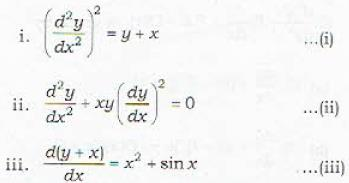are the examples of ordinary differential equations where y is dependent variable and x is independent variable.

QUESTION: 2

### The order of the differential equation is defined as the

Solution:

Definition : The order of the highest ordered derivative involved in a differential equation is called the order of the differential equation. Thus in Q. 1 :
The order of DE(1) = 3
The order of DE(2) = 2
The order of DE(3) = 1

QUESTION: 3

### Which of the following differential equations is of order three?

Solution:

Note that
Order of DE in (a) = 2
Order of DE in (b) = 1
Order of DE in (c) = 3

QUESTION: 4

Which of the following differential equations is of order one?

Solution:

Note that
Order of DE in (a) = 1
Order of DE in (b) = 2
Order of DE in (c) = 2

QUESTION: 5

The degree of a differential equation is defined as the

Solution:

Definition : The degree of a differential equation is defined as the highest power of the highest order derivative involved in the differential, where the equation has been made rational and integral as far as the derivatives are concerned.
degree of DE (1) = 2
degree of DE (2) = 1
degree of DE (3) = 1
An Important Example : Find the degree of the differential equation.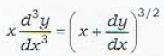In order to rationalize this equation, we first square this equation and get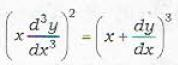Now clearly the degree of the differential equation is 2.
Thus statement (b) gives the correct definition of the degee of a differential equation.

QUESTION: 6

What is the degree of the differential equation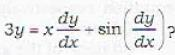Solution:

Degree of this differential equation ean not be defined.

QUESTION: 7

Let m be the order of a differential equation. Then

Solution:

It is clear from the definition that the order of a differential euation is unique.

QUESTION: 8

Let m be the order of a given differential equation. Then

Solution:
QUESTION: 9

Which of the following differential equations is not of degree 1?

Solution:

i. Clearly degree of DE in (a) = 1
ii. DE in (b) must be first squared to make it. rational. Then differential equation becomes.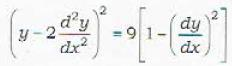or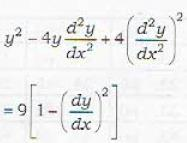so that the highest power of the highest order derivative is 2. Hence its degree is 2.
iii. The differential equations in (c) and (d) must be squared first to make them rational.
Then we find that their degree is 1.
∴ The differential equations in (a), (c) and (d) are each of degree 1 and in (b) is of degree 2.

QUESTION: 10

Which of the following differential equations is of degree 4?

Solution:

Verify that
degree of DE in (a) = 4
degree of DE in (b) = 2
degree of DE in (c) = 2
degree of DE in (d) = 2
Remark : The equations in (a), (c) and (d) are made rational by squaring them.

QUESTION: 11

If n denotes the degree of a given differential equation, then

Solution:

See the definition of the degree of a differential equation.

QUESTION: 12

What is the degree of the differential equation, x3 - 6x3 y3 + 2xy = 0?

Solution:

The degree of an equation that has not more than one variable in each term is the exponent of the highest power to which that variable is raised in the equation. But when more than one variable appears in a term, it is necessary to add the exponents of the variables within a term to get the degree of the equation. Hence, the degree of the equation, x3 - 6x3 y3 + 2xy = 0, is 3 + 3 = 6.

QUESTION: 13

If m and n are the order and degree of the differential equation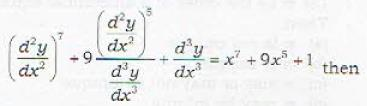Solution:

Rewrite the given differential equation as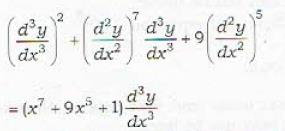Then clearly, its order is 3 and degree is 2.

QUESTION: 14

If  m and n be the order and degree of the differential equation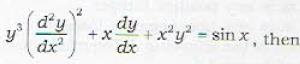Solution:

Here m = n = 2

QUESTION: 15

Which of the following differential equations of first order and second degree?

Solution:

DE (a) is of order 2 and degree 1
DE (b) is of order 1 and degree 2
DE (c) is of order 1 and degree 1
DE (d) is of order 2 and decree 1

QUESTION: 16

The solution of the differential equation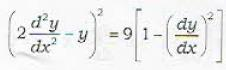is given by

Solution:

Verify by actual substitution of y = sinx and y = cos x in the given differential equation.

QUESTION: 17

Which of the following is a solution of the differential equation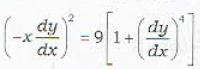Solution:
QUESTION: 18

Which of the following solutions of the differential equation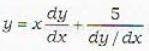is a singular solution?

Solution:

Some Definitions.
General solution : A solution of the differential equation of order n containing n essential arbitrary constants is called a general solution of the differential equation.
Particular solution : A solution which is obtained from the general solution by giving particular values to the arbitrary constants is called a Particular solution.
Singular solution : A solution which can not be obtained from the general solution of the differential equation by any chioice of the n essential arbitrary constants is ealled a Singular solution.
Ex : Consider the differential eauation.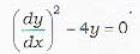..(i)
We have
General solution :    y = (x + c)2
Particular solutions : y = x : For c = 0
y = x + 10 : For c = 10 etc.
Singular solutions : y = 0 Note that y = 0 satisfies the differential equation (i) and can not be obtained from the general solution by giving any value to c.
The given differential equation is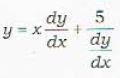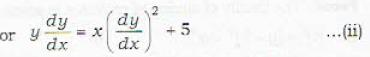Its general solution is given by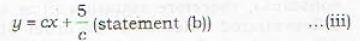But the solution y2 - 20x (verify that it satisfies equation (ii)) but it can not be obtained from the general solution given by (iii) for any value of c. Therefore, y2 -= 20x is a singular solution of differential equation (ii).

QUESTION: 19

A singular solution of the given differential equation is

Solution:
QUESTION: 20

Which one of the following is a differential equation of the family of curves y = Ae2x + Be2x for different values of A and B?

Solution:

We shall explain the method’ of obtaining the differential equation satisfied by a given family of curves.
The method is to eliminate the constants in the family (these constants arc known as the parameters of the family).
Solution : Ex. 1 : Find the differential equation satisfied by
y = cx + c    ...(i)
for different values of c.
Solution : Differentiating (i) we get
dy/dx = c   .....(ii)
Eliminating e from (i) and (ii)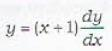Example : 2 : Determine the differential equation whose general solution is
y = Ae2x + Be2x ...(iii)
Solution : Defferendating equation (iii), we get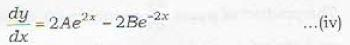Differentiating (iv) again,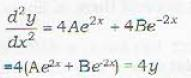∴ Required differential equation is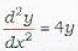Remark : Note that you have to differentiate the given equation of the family of curves as many times as the number of arbitrary constants occuring in the given equation.